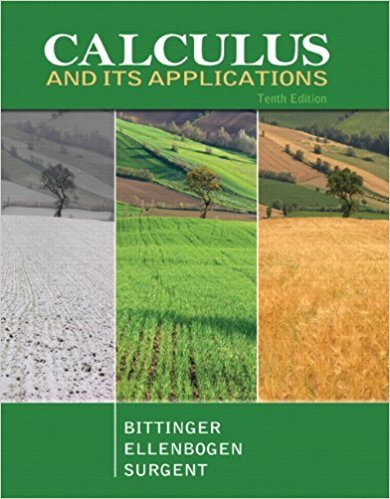×
×

# Solutions for Chapter 5.6: Volume## Full solutions for Calculus and Its Applications | 10th Edition

ISBN: 9780321694331Solutions for Chapter 5.6: Volume

Solutions for Chapter 5.6
4 5 0 410 Reviews
29
2
##### ISBN: 9780321694331

This textbook survival guide was created for the textbook: Calculus and Its Applications, edition: 10. Since 26 problems in chapter 5.6: Volume have been answered, more than 25036 students have viewed full step-by-step solutions from this chapter. This expansive textbook survival guide covers the following chapters and their solutions. Calculus and Its Applications was written by and is associated to the ISBN: 9780321694331. Chapter 5.6: Volume includes 26 full step-by-step solutions.

Key Calculus Terms and definitions covered in this textbook

If u = v and w = z , then u + w = v + z

• Axis of symmetry

See Line of symmetry.

• Central angle

An angle whose vertex is the center of a circle

• Demand curve

p = g(x), where x represents demand and p represents price

• Dihedral angle

An angle formed by two intersecting planes,

• Discriminant

For the equation ax 2 + bx + c, the expression b2 - 4ac; for the equation Ax2 + Bxy + Cy2 + Dx + Ey + F = 0, the expression B2 - 4AC

• Equation

A statement of equality between two expressions.

• Equilibrium point

A point where the supply curve and demand curve intersect. The corresponding price is the equilibrium price.

• General form (of a line)

Ax + By + C = 0, where A and B are not both zero.

• Hyperboloid of revolution

A surface generated by rotating a hyperbola about its transverse axis, p. 607.

• Identity matrix

A square matrix with 1’s in the main diagonal and 0’s elsewhere, p. 534.

• Local extremum

A local maximum or a local minimum

• Monomial function

A polynomial with exactly one term.

• Polar coordinate system

A coordinate system whose ordered pair is based on the directed distance from a central point (the pole) and the angle measured from a ray from the pole (the polar axis)

• Probability function

A function P that assigns a real number to each outcome O in a sample space satisfying: 0 … P1O2 … 1, P12 = 0, and the sum of the probabilities of all outcomes is 1.

A function that can be written in the form ƒ(x) = ax 2 + bx + c, where a, b, and c are real numbers, and a ? 0.

A procedure for fitting a quadratic function to a set of data.

• Row operations

See Elementary row operations.

• Slope-intercept form (of a line)

y = mx + b

×